# 10-327/Classnotes for Monday September 27

See some blackboard shots at BBS/10_327-100927-142655.jpg.

 Dror's notes above / Student's notes below

Here are some lecture notes..

Xwbdsb 20:26, 27 September 2010 (EDT)

• Question 1: Dror you said in class the set of permutations of 0's and 1's could be mapped "bijectively" onto the unit interval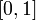$[0,1]$ and hence is not countable. Is it true that every real number in the unit interval has more than one binary expansion? Is it possible to map the set of all permutations onto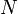$N$ union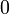${0}$? (the first number stands for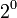$2^0$, second stands for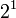$2^1$, etc.) -Kai Xwbdsb 20:37, 27 September 2010 (EDT)
• Except for the "dyadic rationals", the numbers of the form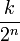$\frac{k}{2^n}$, real numbers have a unique binary expansion, and there is only a countable set of dyadic rationals. Also, we are talking about sequences of 0s and 1s, not "permutations". And just like decimals, binary number may have a "decimal point", where the (finite) part of the sequence ahead of the point represents the integer part of the number and the (possibly infinite) sequence after the point represents the fractional part. Drorbn 20:51, 27 September 2010 (EDT)
• So here both k and n are integers? Also what if I don't use decimal point to represent a sequence of 0s and 1s just mapping the sequence into the natural numbers? Then wouldn' the set be countable? -Kai Xwbdsb 09:06, 28 September 2010 (EDT)
• Yes,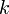$k$ and$n$ here are integers. To represent integers, you use finite sequences of 0s and 1s, and there are countably many of those. To represent real numbers in$[0,1]$ you use infinite sequences of 0s and 1s, and there are uncountably many of those. Drorbn 09:39, 28 September 2010 (EDT)
• Question 2: Suppose we have a collection of sets which is closed under intersection. Does it mean that it is closed under arbitrary intersecions(i.e. uncountable intersections?) Also, suppose this set satisfies the condition: for any A,B in the set, A intersect B is in the set. What can mathematical induction give us? A.the set is closed under finite intersections B.the set is closed under countable intersections C.the set is closed under arbitrary intersections. And Why? -Kai
• Induction will only go to finite numbers. Drorbn 20:51, 27 September 2010 (EDT)
• If n = 1 is true, n=k is true implies n=k+1 is true. Why wouldn't this logic imply that n=infinity is true?-Kai Xwbdsb 09:12, 28 September 2010 (EDT)
• No, it does not imply that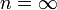$n=\infty$ is true. Yet I cannot give a full review of induction here; you may want to read Wikipedia: Mathematical Induction and/or come to my office hours. Drorbn 09:40, 28 September 2010 (EDT)

D 12:28, 2 October 2010 (EDT) Note on clopen (closed and open) set: In ANY topological space, an empty set is clopen. Similarly, the whole set is clopen. However it is not true that any clopen set is trivial (although it is true in a connected space, since it cannot be represented by two disjoint open sets). For example, consider Q = {all rational numbers} and its subset A = {all rational numbers smaller than pi}. Then the boundary of A is empty in Q, because pi is not in Q. Hence A is clopen in Q. Of course, A is not open nor closed in R.